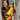# Python super() vs Base.__init__ Method

July 19, 2021 | 4 mins

When defining a subclass, there are different ways to call the `__init__` method of a parent class. Let’s start with a base class and go through each of these methods.

For this blog, it’s better that you open a `sample.py` python file and follow along.

``````class Base(object):
def __init__(self):
print "Base created"``````

### Method 1 :: Using Parent Reference Directly

``````class ChildA(Base):
def __init__(self):
Base.__init__(self)
print ("Child A initlaized")``````

### Method 2:: Using Super with child class

``````class ChildB(Base):
def __init__(self):
print ("Child B initlaized")
super(ChildB, self).__init__()``````

### Method 3:: Using the super method

``````class ChildC(Base):
def __init__(self):
super().__init__()
print ("Child C initlaized")``````

## Questions

1. What are the pros and cons of each method?
2. Is there one single right way to do this?

When you run this code as a single Python script, initializing child classes A, B, and C., You will notice absolutely no difference.

``````cA = ChildA()
cB = ChildB()
cC = ChildC()``````

1. As of Python3 `super()` is same as `super(ChildB, self).__init__()`. That rules out one of the three methods.
2. To compare `Base.__init__(self)` and `super().__init__()` we need multiple Inheritance. Consider the following snippet
``````class Base1:
def __init__(self):
print ("Base 1 created")
super().__init__()

class Base2:
def __init__(self):
print ("Base 2 created")
super().__init__()

class A1(Base1, Base2):
def __init__(self):
super().__init__()
print ("Child A1 initlaized")

class A2(Base2, Base1):
def __init__(self):
super().__init__()
print ("Child A2 initlaized")

a1 = A1()

print ("\n\n")
a2 = A2()``````

On running the above snippet, we get the following Output.

``````Base 1 created
Base 2 created
Child A1 initialized

Base 2 created
Base 1 created
Child A2 initialized``````

In the case of class `A1(Base1, Base2)` `Base1` is initialized first, followed by `Base2`. It’s the inverse for class `A2`. We can conclude that the methods are called based on the order of specification.

1. When you use the `Base1.__init__()` method, you lose out on this feature of Python
2. When you introduce new hierarchies, renaming the classes will become a nightmare

So how does Python know which function to call first, introducing MRO(Method Resolution Order)

## Method Resolution Order

Method Resolution Order(MRO) denotes the way a programming language resolves a method or attribute.

In the case of single inheritance, the attribute is searched only at a single level, with multiple inheritance Python interpreter looks for the attribute in itself then its parents in the order of inheritance. In case of `A1 -> Base 1 -> Base 2`

One can use the `mro` function to find the method resolution order of any particular class.

``print (A2.mro())``

** Output**

``[<class '__main__.A2'>, <class '__main__.Base2'>, <class '__main__.Base1'>, <class 'object'>]``

## The Last Punch

Comment out the super calls in base class and check the ouput of your script.

``````class Base1:
def __init__(self):
print ("Base 1 created")
# super().__init__()

class Base2:
def __init__(self):
print ("Base 2 created")
# super().__init__()``````

What do you see?

``````Base 1 created
Child A1 initlaized
Base 2 created
Child A2 initlaized

[<class '__main__.A2'>, <class '__main__.Base2'>, <class '__main__.Base1'>, <class 'object'>]``````

Inspite of having `Base1` and `Base2` in the `mro` list, `mro` won’t resolve the order unless the `super()` function is propogated all the way up to the base class i.e., Python propogates the search for the attribute only until it finds one. Comment the init method in Base1 and see for yourself

``````class Base1:
pass
# def __init__(self):
#     self.prop1 = "Base 1"
#     self.prop11 = "Base 11"
#     print ("Base 1 created")
#     # super().__init__()
``````

Output

Since Python can’t find the `__init__` method in `Base1` it checks `Base2` before sending it all the way to `object` class

``````Base 2 created
Child A1 initlaized

Base 2 created
Child A2 initlaized
[<class '__main__.A2'>, <class '__main__.Base2'>, <class '__main__.Base1'>, <class 'object'>]``````

People ask me why I love Python so much, it’s not because Python is simple and easy. It is all these things Python does to make things easy for us, sometimes a little hard too :)

## Join the TheLearningDev Community!

Go from learning Python to Building ProjectsWritten by Bhavani Ravi Code | Speak | Read | Write | Teach Technology